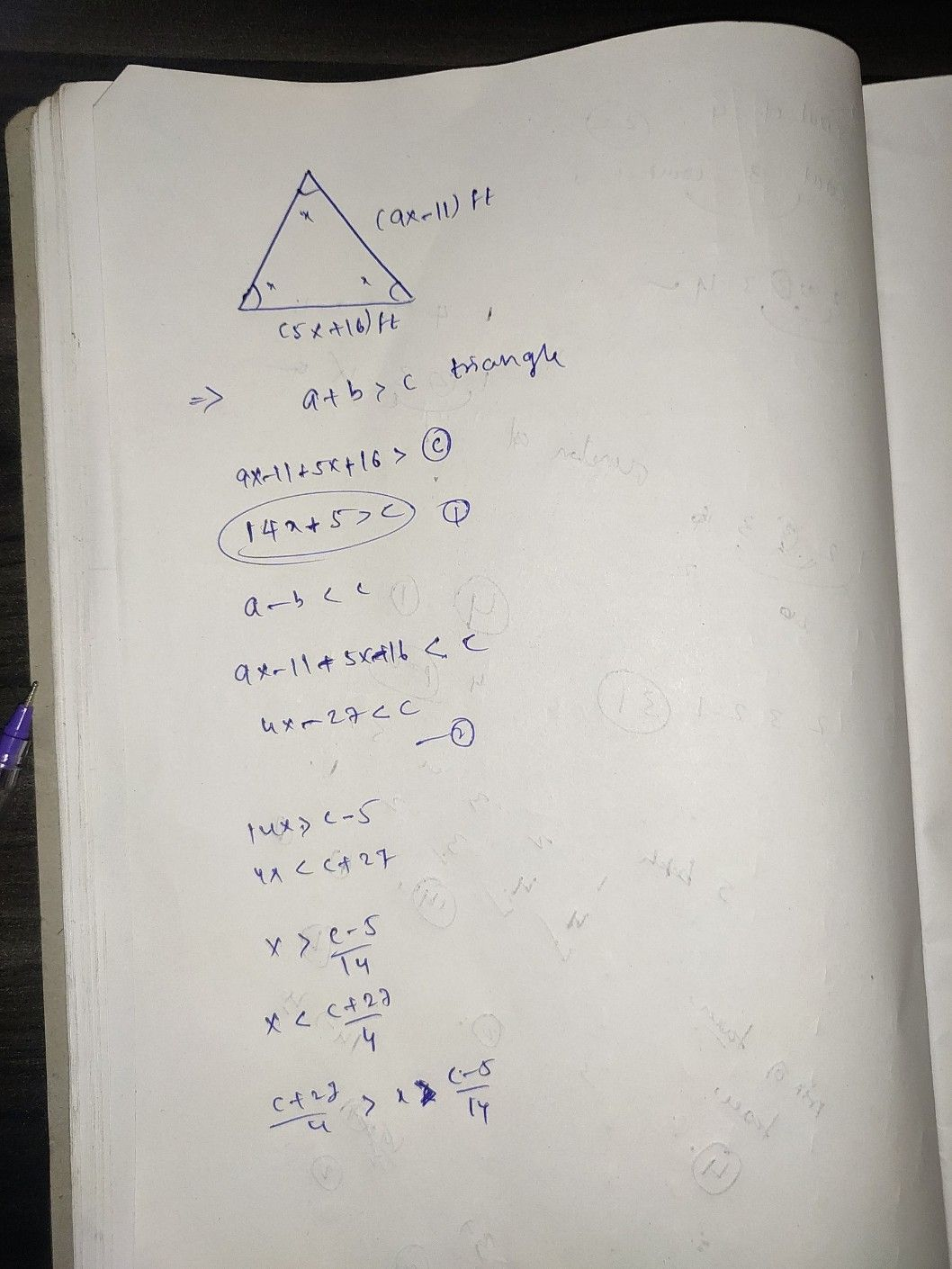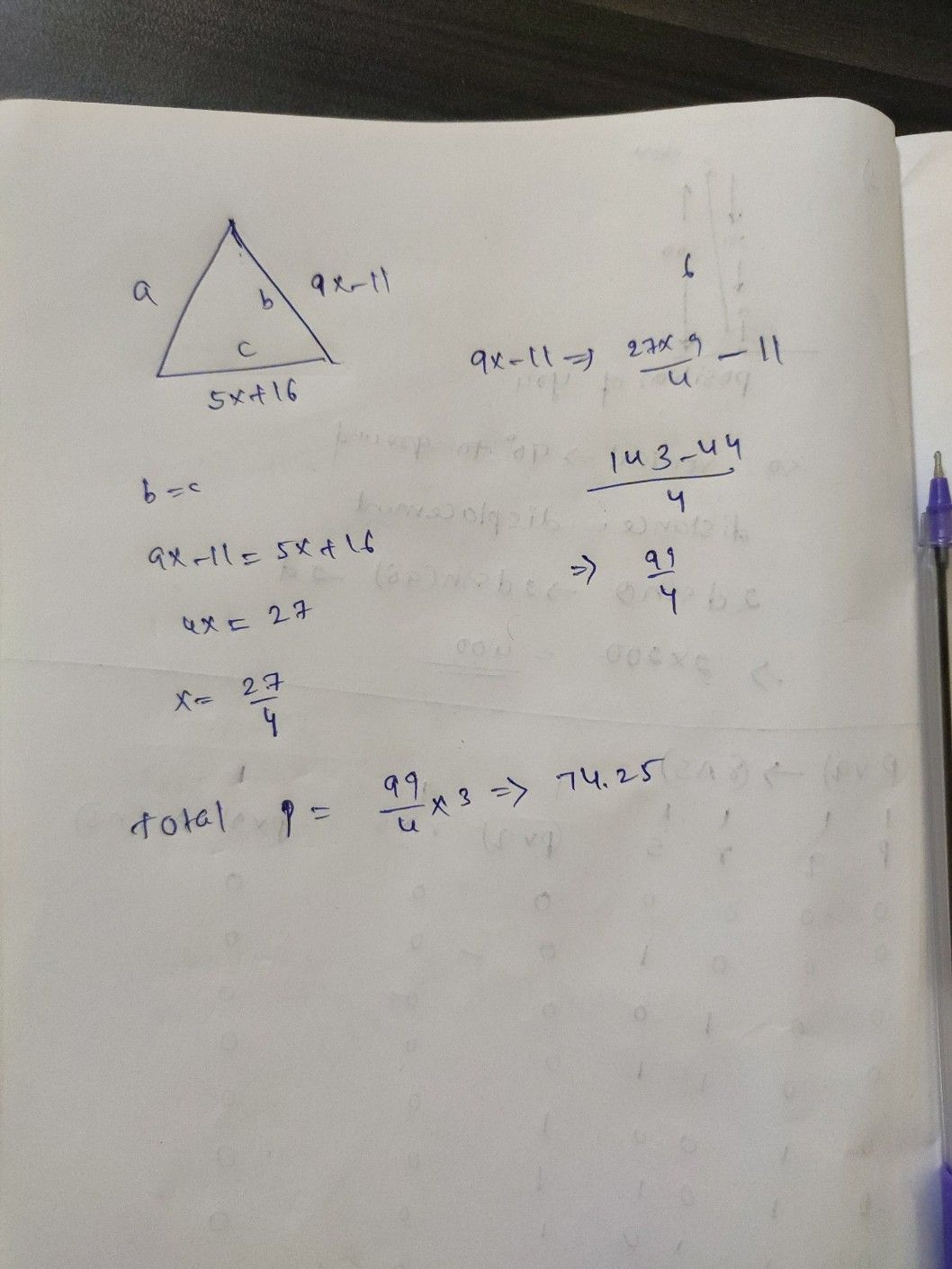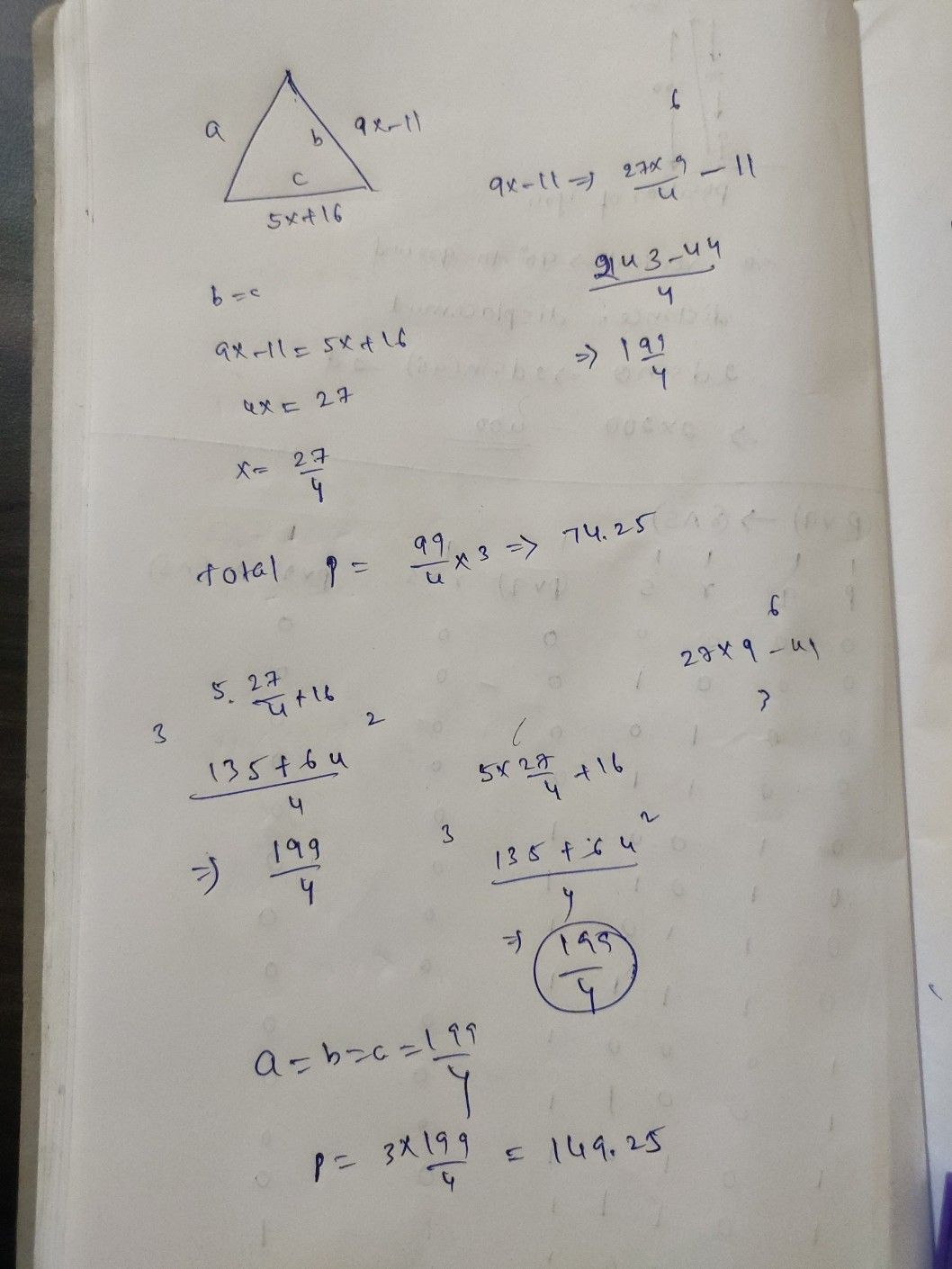Symbol
ProblemFind the perimeter of the triangle. $\left(9x-11\right)$ $\pi$ $\left(5x+16\right)$ $\pi$ $P=$ type your answer... units
Geometry
SolutionQanda teacher - ManoharStudent
What’s the ANSWR excatly